# The present application concerns the relation between per capita consumption (LogConsumption), per capita income

 Page 1/10 Date 23.04.2018 Size 1.07 Mb.

## Problem 1

The present application concerns the relation between per capita consumption (LogConsumption), per capita income (LogIncome) and per capita wealth (LogWealth). As the names of the variables indicate, the original variables have been transformed by taking logarithm. On the basis of quarterly time series data for these variables, running from 1967- 2 until 2004- 4, we wish to find out if there is long run relationship between these variables. You will find attached description of the data, tests and regression outputs that will help you to answer the questions.
Question 1.1

In analysing time series data in econometrics one often transforms the original data by taking logarithm. Can you give some reasons why this is a useful approach.

The unit root tests use the Augmented Dickey-Fuller test procedure. This test is based on the fact that an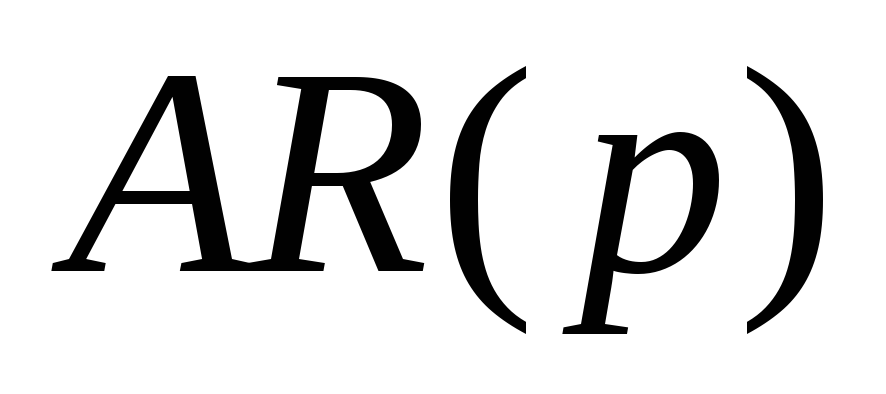process:
(1)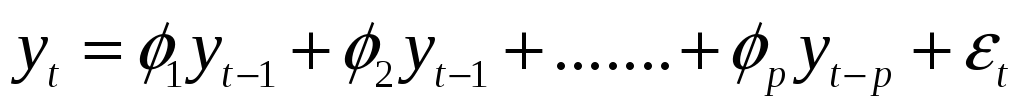can, equivalently be written as:
(2)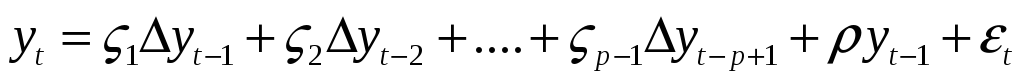Question 1.2

Give an explanation of the Augmented Dickey-Fuller test.

The attached regression and test results are edited outputs obtained by the PcGive program.
In the output of the ADF tests given at the end of the problem set, the first column D-lag refers to the number of lags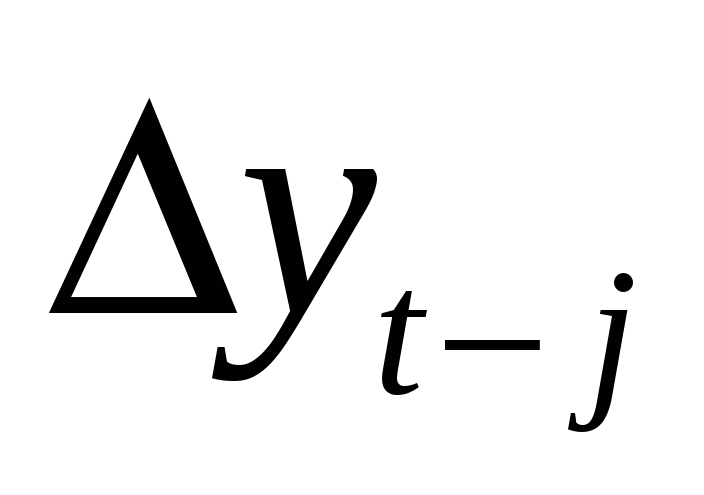that are included in the regression, the column t-adf shows the values of the test statistic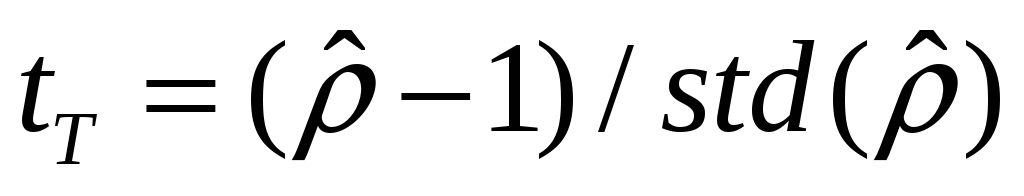(wheredenotes the standard deviation of) , the column denoted asY_1 shows the estimates of the parameter, the column t-DY_lag reports the values of the statistic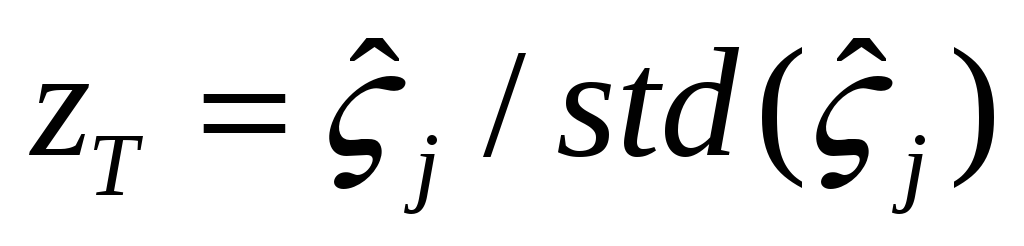, and finally, the column t-prob shows the corresponding probability value.

For each variable we report three tables referring to the different specifications of the regression equation and the different null hypothesis.